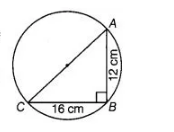# If AB = 12 cm, BC = 16 cm and AB`
Question:

If AB = 12 cm, BC = 16 cm and AB is perpendicular to BC, then the radius of the circle passing through the points A, B and C is

(a) 6 cm

(b) 8 cm

(c) 10 cm

(d) 12 cm

Solution:

(c) Given, $A B=12 \mathrm{~cm}$ and $B C=16 \mathrm{~cm}$

In a circle, $B C \perp A B$, it means that $A C$ will be a diameter of circle.[diameter of a circle subtends a right angle to the circle]

Use Pythagoras theorem in right angled $\triangle A B C$,

$A C^{2}=A B^{2}+B C^{2}$

$\Rightarrow$ $A C^{2}=(12)^{2}+(16)^{2}$

$\Rightarrow$ $A C^{2}=144+256 \Rightarrow A C^{2}=400$

$\Rightarrow$ $A C=\sqrt{400}=20 \mathrm{~cm}$

[taking positive square root, because diameter is always positive]

$\therefore \quad$ Radius of circle $=\frac{1}{2}(A C)=\frac{1}{2} \times 20=10 \mathrm{~cm}$

Hence, the radius of circle is $10 \mathrm{~cm}$.